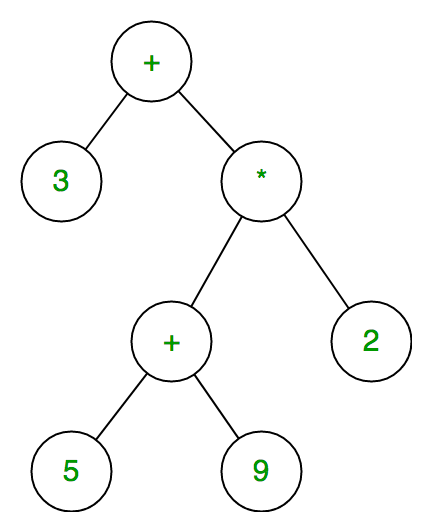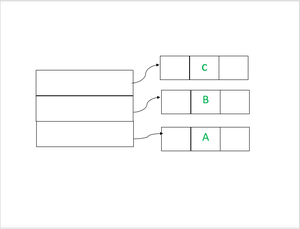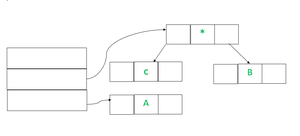# Expression Tree

The expression tree is a binary tree in which each internal node corresponds to the operator and each leaf node corresponds to the operand so for example expression tree for 3 + ((5+9)*2) would be:Inorder traversal of expression tree produces infix version of given postfix expression (same with postorder traversal it gives postfix expression)

Evaluating the expression represented by an expression tree:

```Let t be the expression tree
If  t is not null then
If t.value is operand then
Return  t.value
A = solve(t.left)
B = solve(t.right)

// calculate applies operator 't.value'
// on A and B, and returns value
Return calculate(A, B, t.value)```

Construction of Expression Tree:

Now For constructing an expression tree we use a stack. We loop through input expression and do the following for every character.

1. If a character is an operand push that into the stack
2. If a character is an operator pop two values from the stack make them its child and push the current node again.

In the end, the only element of the stack will be the root of an expression tree.

Examples:

```Input:  A B C*+ D/
Output: A + B * C / D```

The first three symbols are operands, so create tree nodes and push pointers to them onto a stack as shown below.In the Next step, an operator ‘*’ will going read, so two pointers to trees are popped, a new tree is formed and a pointer to it is pushed onto the stackIn the Next step,  an operator ‘+’ will read, so two pointers to trees are popped, a new tree is formed and a pointer to it is pushed onto the stack.

A3f.png

Similarly, as above cases first we push ‘D’ into the stack and then in the last step first, will read ‘/’ and then as previous step topmost element will pop out and then will be right subtree of root  ‘/’ and other nodes will be right subtree.

Final Constructed Expression Tree is:

A4f.png

Below is the code of the above approach:

Recommended Practice

Below is the implementation of the above approach:

## C++

 `// C++ program for expression tree` `#include ` `using` `namespace` `std;` `class` `node {` `public``:` `    ``char` `value;` `    ``node* left;` `    ``node* right;` `    ``node* next = NULL;` `    ``node(``char` `c)` `    ``{` `        ``this``->value = c;` `        ``left = NULL;` `        ``right = NULL;` `    ``}` `    ``node()` `    ``{` `        ``left = NULL;` `        ``right = NULL;` `    ``}` `    ``friend` `class` `Stack;` `    ``friend` `class` `expression_tree;` `};` `class` `Stack {` `    ``node* head = NULL;`   `public``:` `    ``void` `push(node*);` `    ``node* pop();` `    ``friend` `class` `expression_tree;` `};` `class` `expression_tree {` `public``:` `    ``void` `inorder(node* x)` `    ``{` `        ``// cout<<"Tree in InOrder Traversal is: "<left);` `            ``cout << x->value << ``"  "``;` `            ``inorder(x->right);` `        ``}` `    ``}` `};`   `void` `Stack::push(node* x)` `{` `    ``if` `(head == NULL) {` `        ``head = x;` `    ``}` `    ``// We are inserting here nodes at the top of the stack [following LIFO principle]` `    ``else` `{` `        ``x->next = head;` `        ``head = x;` `    ``}` `}` `node* Stack::pop()` `{` `    ``// Popping out the top most[ pointed with head] element` `    ``node* p = head;` `    ``head = head->next;` `    ``return` `p;` `}` `int` `main()` `{` `    ``string s = ``"ABC*+D/"``;` `    ``// If you  wish take input from user:` `    ``//cout << "Insert Postorder Expression: " << endl;` `    ``//cin >> s;` `    ``Stack e;` `    ``expression_tree a;` `    ``node *x, *y, *z;` `    ``int` `l = s.length();` `    ``for` `(``int` `i = 0; i < l; i++) {` `        ``// if read character is operator then popping two` `        ``// other elements from stack and making a binary` `        ``// tree` `        ``if` `(s[i] == ``'+'` `|| s[i] == ``'-'` `|| s[i] == ``'*'` `            ``|| s[i] == ``'/'` `|| s[i] == ``'^'``) {` `            ``z = ``new` `node(s[i]);` `            ``x = e.pop();` `            ``y = e.pop();` `            ``z->left = y;` `            ``z->right = x;` `            ``e.push(z);` `        ``}` `        ``else` `{` `            ``z = ``new` `node(s[i]);` `            ``e.push(z);` `        ``}` `    ``}` `    ``cout << ``" The Inorder Traversal of Expression Tree: "``;` `    ``a.inorder(z);` `    ``return` `0;` `}`

## C

 `#include ` `#include `   `/* A binary tree node has data, pointer to left child` `   ``and a pointer to right child */` `struct` `node {` `    ``char` `data;` `    ``struct` `node* left;` `    ``struct` `node* right;` `    ``struct` `node* next;` `};` ` ``struct` `node *head=NULL;` `/* Helper function that allocates a new node with the` `   ``given data and NULL left and right pointers. */` `struct` `node* newNode(``char` `data)` `{` `    ``struct` `node* node` `        ``= (``struct` `node*)``malloc``(``sizeof``(``struct` `node));` `    ``node->data = data;` `    ``node->left = NULL;` `    ``node->right = NULL;` `    ``node->next = NULL;` `    `  `    ``return` `(node); ` `}` `void` `printInorder(``struct` `node* node)` `{` `    ``if` `(node == NULL)` `        ``return``;` `    ``else``{` `    ``/* first recur on left child */` `    ``printInorder(node->left);`   `    ``/* then print the data of node */` `    ``printf``(``"%c "``, node->data);`   `    ``/* now recur on right child */` `    ``printInorder(node->right);` `    ``}` `}`   `void` `push(``struct` `node* x)` `{` `    ``if``(head==NULL)` `    ``head = x;` `    ``else` `    ``{` `        ``(x)->next = head;` `        ``head  = x;` `    ``}` `    ``// struct node* temp;` `    ``// while(temp!=NULL)` `    ``// {` `    ``//     printf("%c ", temp->data);` `    ``//     temp = temp->next;` `    ``// }` `}` `struct` `node* pop()` `{` `    ``// Popping out the top most[ pointed with head] element` `    ``struct` `node* p = head;` `    ``head = head->next;` `    ``return` `p;` `}` `int` `main()` `{` `    ``char` `s[] = {``'A'``,``'B'``,``'C'``,``'*'``,``'+'``,``'D'``,``'/'``};` `    ``int` `l = ``sizeof``(s) / ``sizeof``(s) ;` `    ``struct` `node *x, *y, *z;` `    ``for` `(``int` `i = 0; i < l; i++) {` `        ``// if read character is operator then popping two` `        ``// other elements from stack and making a binary` `        ``// tree` `        ``if` `(s[i] == ``'+'` `|| s[i] == ``'-'` `|| s[i] == ``'*'` `            ``|| s[i] == ``'/'` `|| s[i] == ``'^'``) {` `            ``z = newNode(s[i]);` `            ``x = pop();` `            ``y = pop();` `            ``z->left = y;` `            ``z->right = x;` `            ``push(z);` `        ``}` `        ``else` `{` `            ``z = newNode(s[i]);` `            ``push(z);` `        ``}` `    ``}` `    ``printf``(``" The Inorder Traversal of Expression Tree: "``);` `    ``printInorder(z);` `    ``return` `0;` `}`

## Java

 `import` `java.util.Stack;`   `class` `Node{` `    ``char` `data;` `    ``Node left,right;` `    ``public` `Node(``char` `data){` `        ``this``.data = data;` `        ``left = right = ``null``;` `    ``}` `}`   `public` `class` `Main {` `    ``public` `static` `boolean` `isOperator(``char` `ch){` `        ``if``(ch==``'+'` `|| ch==``'-'``|| ch==``'*'` `|| ch==``'/'` `|| ch==``'^'``){` `            ``return` `true``;` `        ``}` `        ``return` `false``;` `    ``}` `    ``public` `static` `Node expressionTree(String postfix){` `        ``Stack st = ``new` `Stack();` `        ``Node t1,t2,temp;`   `        ``for``(``int` `i=``0``;i

## Python3

 `class` `Node:` `    ``def` `__init__(``self``, value``=``None``, left``=``None``, right``=``None``, ``next``=``None``):` `        ``self``.value ``=` `value` `        ``self``.left ``=` `left` `        ``self``.right ``=` `right` `        ``self``.``next` `=` `next`   `class` `Stack:` `    ``def` `__init__(``self``):` `        ``self``.head ``=` `None`   `    ``def` `push(``self``, node):` `        ``if` `not` `self``.head:` `            ``self``.head ``=` `node` `        ``else``:` `            ``node.``next` `=` `self``.head` `            ``self``.head ``=` `node`   `    ``def` `pop(``self``):` `        ``if` `self``.head:` `            ``popped ``=` `self``.head` `            ``self``.head ``=` `self``.head.``next` `            ``return` `popped` `        ``else``:` `            ``raise` `Exception(``"Stack is empty"``)`   `class` `ExpressionTree:` `    ``def` `inorder(``self``, x):` `        ``if` `not` `x:` `            ``return` `        ``self``.inorder(x.left)` `        ``print``(x.value, end``=``" "``)` `        ``self``.inorder(x.right)`   `def` `main():` `    ``s ``=` `"ABC*+D/"` `    ``stack ``=` `Stack()` `    ``tree ``=` `ExpressionTree()` `    ``for` `c ``in` `s:` `        ``if` `c ``in` `"+-*/^"``:` `            ``z ``=` `Node(c)` `            ``x ``=` `stack.pop()` `            ``y ``=` `stack.pop()` `            ``z.left ``=` `y` `            ``z.right ``=` `x` `            ``stack.push(z)` `        ``else``:` `            ``stack.push(Node(c))` `    ``print``(``"The Inorder Traversal of Expression Tree: "``, end``=``"")` `    ``tree.inorder(stack.pop())`   `if` `__name__ ``=``=` `"__main__"``:` `    ``main()`

## C#

 `using` `System;` `using` `System.Collections.Generic;`     `class` `Node{` `    ``public` `char` `data;` `    ``public` `Node left,right;` `    ``public` `Node(``char` `data){` `        ``this``.data = data;` `        ``left = right = ``null``;` `    ``}` `}`   `public` `class` `GFG {` `    ``public` `static` `bool` `isOperator(``char` `ch){` `        ``if``(ch==``'+'` `|| ch==``'-'``|| ch==``'*'` `|| ch==``'/'` `|| ch==``'^'``){` `            ``return` `true``;` `        ``}` `        ``return` `false``;` `    ``}` `    ``static` `Node expressionTree(String postfix){` `        ``Stack st = ``new` `Stack();` `        ``Node t1, t2, temp;`   `        ``for``(``int` `i = 0; i < postfix.Length; i++)` `        ``{` `            ``if``(!isOperator(postfix[i])){` `                ``temp = ``new` `Node(postfix[i]);` `                ``st.Push(temp);` `            ``}` `            ``else``{` `                ``temp = ``new` `Node(postfix[i]);`   `                ``t1 = st.Pop();` `                ``t2 = st.Pop();`   `                ``temp.left = t2;` `                ``temp.right = t1;`   `                ``st.Push(temp);` `            ``}`   `        ``}` `        ``temp = st.Pop();` `        ``return` `temp;` `    ``}` `    ``static` `void` `inorder(Node root){` `        ``if``(root == ``null``) ``return``;`   `        ``inorder(root.left);` `        ``Console.Write(root.data);` `        ``inorder(root.right);` `    ``}` `    ``public` `static` `void` `Main(String[] args)` `    ``{` `        ``String postfix = ``"ABC*+D/"``;`   `        ``Node r = expressionTree(postfix);` `        ``inorder(r);` `    ``}` `}`   `// This code is contributed by 29AjayKumar`

## Javascript

 `// Javascript code for the above approach` `class Node {` `    ``constructor(value = ``null``, left = ``null``, right = ``null``, next = ``null``) {` `        ``this``.value = value;` `        ``this``.left = left;` `        ``this``.right = right;` `        ``this``.next = next;` `    ``}` `}`   `class Stack {` `    ``constructor() {` `        ``this``.head = ``null``;` `    ``}`   `    ``push(node) {` `        ``if` `(!``this``.head) {` `            ``this``.head = node;` `        ``} ` `        ``else` `        ``{` `         ``// We are inserting here nodes at ` `         ``// the top of the stack [following LIFO principle]` `            ``node.next = ``this``.head;` `            ``this``.head = node;` `        ``}` `    ``}`   `    ``pop() {` `        ``if` `(``this``.head) {` `            ``let popped = ``this``.head;` `            ``this``.head = ``this``.head.next;` `            ``return` `popped;` `        ``} ``else` `{` `            ``throw` `new` `Error(``"Stack is empty"``);` `        ``}` `    ``}` `}`   `class ExpressionTree {` `    ``inorder(x) {` `        ``if` `(!x) {` `            ``return``;` `        ``}` `        ``this``.inorder(x.left);` `        ``console.log(x.value+``" "``);` `        ``this``.inorder(x.right);` `    ``}` `}`     `    ``let s = ``"ABC*+D/"``;` `    ``let stack = ``new` `Stack();` `    ``let tree = ``new` `ExpressionTree();` `    ``for` `(let c of s) {` `        ``if` `(c === ``"+"` `|| c === ``"-"` `|| c === ``"*"` `|| c === ``"/"` `|| c === ``"^"``) {` `            ``let z = ``new` `Node(c);` `            ``let x = stack.pop();` `            ``let y = stack.pop();` `            ``z.left = y;` `            ``z.right = x;` `            ``stack.push(z);` `        ``} ``else` `{` `            ``stack.push(``new` `Node(c));` `        ``}` `    ``}` `    ``console.log(``"The Inorder Traversal of Expression Tree: "``);` `    ``tree.inorder(stack.pop());`   `// This code is contributed by lokeshpotta20.`

Output

` The Inorder Traversal of Expression Tree: A  +  B  *  C  /  D  `

Time complexity: O(n)
Auxiliary space: O(n)

Feeling lost in the world of random DSA topics, wasting time without progress? It's time for a change! Join our DSA course, where we'll guide you on an exciting journey to master DSA efficiently and on schedule.
Ready to dive in? Explore our Free Demo Content and join our DSA course, trusted by over 100,000 geeks!

Previous
Next